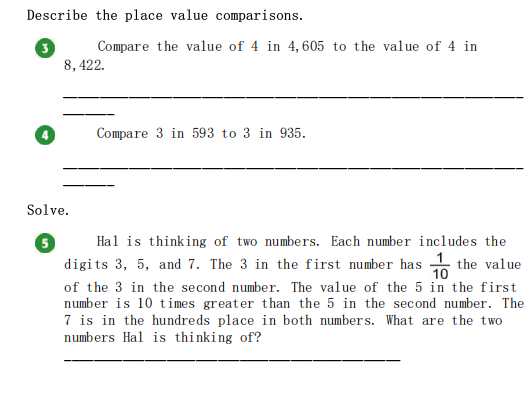### ¿Todavía tienes preguntas de matemáticas?

Pregunte a nuestros tutores expertos
Algebra
Pregunta(5) Hal is thinking of two numbers. Each number includes the digits $$3,5$$ , and $$7$$ . The $$3$$ in the first number has $$\frac { 1 } { 10 }$$ the value of the $$3$$ in the second number. The value of the $$5$$ in the first number is $$10$$ times greater than the $$5$$ in the second number. The $$7$$ is in the hundreds place in both numbers. What are the two numbers Hal is thinking of?